# Room dimensions

Room dimensions are 5m and 3.5m. Room height is 2.85m. Paint the room (even with the ceiling). There will be 2 layers. Doors and windows have a total of 2.5 m2. One box of paint is enough for 6m2. How many boxes of paint are needed? How much do we pay if 1 box costs 5 €?

n =  22
x =  110 Eur

### Step-by-step explanation: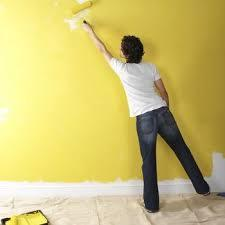Did you find an error or inaccuracy? Feel free to write us. Thank you!#### You need to know the following knowledge to solve this word math problem:

We encourage you to watch this tutorial video on this math problem:

## Related math problems and questions:

• Jared's room paintingJared wants to paint his room. The room's dimensions are 12 feet by 15 feet, and the walls are 9 feet high. Two windows measure 6 feet by 5 feet each. There are two doors, whose dimensions are 30 inches by 6 feet each. If a gallon of paint covers approxim
• Painter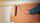How many euros we will pay for repainting the room shaped cuboid with a length of 4.5 meters, width of 2.5 meters and a height of 3 meters, if for 1 m2 with paint we pay € 1.5?
• The roomThe room has a cuboid shape with dimensions: length 50m and width 60dm and height 300cm. Calculate how much this room will cost paint (a floor is not painted) if the window and door area is 15% of the total area and 1m2 cost 15 euro.
• If one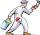If one litre of pant covers an area of 5 m2 how much paint is needed to cover: a) rectangular swimming pool With dimensions 4m x 3m x 2.5m (the Inside walls and the floor only) b) the Inside walls and floor of a cylindrical reservoir with diameter 3m and
• Painting a hutIt is necessary to paint the exterior walls of hut whose layout is a rectangle of 6.16 m x 8.78 m wall height is 2.85 meters. Cottage has five rectangular windows; three have dimensions of 1.15 m x 1.32 m and two 0,45 m x 0.96 m. How many m2 is necessary
• Two sides paintThe door has the shape of a rectangle with dimensions of 260cm and 170cm. How many cans of paint will be needed to paint this door if one can of paint cover 2m2 of the area? We paint the doors on both sides.
• PaintingThe room is long 50 meters and wide 60dm and 300 cm high. Calculate how much it will cost painting if area of windows and doors is 15% of the total area. One square meter cost 50cents.
• PaintingTo paint the pool with dimensions: 2 meters depth, 3m x 4m we bought paint to 50 meters square. How many "paint" will be a waste?
• Insulate houseThe property owner wants to insulate his house. The house has these dimensions 12, and 12 m is 15 m high. The windows have 6 with dimensions 170 and 150 cm. Entrance doors are 250 and 170 cm in size. How many square meters of polystyrene does he need?
• FlowerpotThe block-shaped flowerpot has external dimensions: length 1.25 m, width 10 cm, and height 11 cm. The thickness of the boards from which it is made is 0.8 cm. How many liters of soil is needed to fill it 1 cm below the top edge? What surface do we have to
• Concrete pipe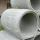How much will cost cover a 6 m long concrete pipe with an outer radius 1.5 m and inner radius 0.8 meters if 1 m2 paint costs 24 €.
• The wall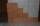The wall in the bathroom has dimensions of 2.8 m and 2.6 m. If we would like to cover this wall to a height of 1.6 m. How many square meters of tiles do we need to buy?
• Wooden box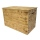The block-shaped box was placed on the ground, leaving a rectangular print with 3 m and 2 m. When flipped over to another wall, a print with dimensions of 0.5 m and 3 m remained in the sand. What is the volume of the wooden box?
• Pool tilesThe pool is 25m long, 10m wide, and 160cm deep. How many m2 of tiles will be needed on the walls and the pool? How many tiles are needed when 1 tile has a square shape with a 20cm side? How much does it cost when 1m2 of tiles costs 258 Kc?
• Paint cansHow many paint cans do we need to paint the floor in two rooms with dimensions of 6.8m x 4.5m and 6m x3.8m? One can are for 6m².
• Wall painting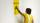The wall is 4 meters wide and 2 meters high. The window in the wall has dimensions of 2x1,8 meters. How many liters of color is needed to paint two-layer these walls, if the 1 m2 needs 1 liters of paint?
• How many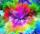How many cans of blue paint need to be bought if the interior of the garden pool, which is 5 m long, 3 m wide and 1 m deep, is to be painted? There is 1 kg of paint in each can. One can is enough for 8 m2 of area.